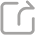分享至QQ空间 分享至朋友圈 分享至新浪微博

### Hydrodynamic model of semiconductors with sonic boundary: structure stability and quasi-neutral limit

This talk is concerned with the structural stability of subsonic steady states and quasi-neutral limit to one-dimensional steady hydrodynamic model of semiconductors in the form of Euler-Poisson equations with degenerate boundary, a difficult case caused by the boundary layers and degeneracy.  We first prove that the subsonic steady states are structurally stable, once the perturbation of doping profile is small enough. To overcome the singularity at the sonic boundary, we introduce an optimal weight in the energy edtimates. For the quadi-neutral limit, we establish a so-called convexity structure of the sequence of subsonic-sonic solutions near the boundary domains in this limit process, which efficiently overcomes the degenerate effect.  On this account, we first show the strong convergence in $L^2$ norm with the order $O(\lambda^\frac{1}{2})$  for the Debye length $\lambda$ when the doping profile is continuous. Then we derive the uniform error estimates in $L^\infty$ norm with the order $O(\lambda)$ when the doping profile has higher regularity.  This talk is based on two recent research papers published in SIAM J. Math. Anal. (2023).